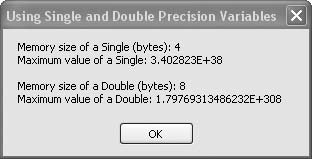# Recipe 6.5. Using Single- and Double-Precision Variables

#### Problem

You want to use floating-point numbers but aren't sure if you should use Singles or Doubles.

#### Solution

Sample code folder: Chapter 06\SingleDouble

Choose the most appropriate variable type based on the range and precision of numbers it can hold and on its memory footprint.

#### Discussion

To help you understand the capabilities of Single and Double variables, the following sample code uses several useful properties and functions to display information about them:

` Dim result As New System.Text.StringBuilder Dim maxSingle As Single = Single.MaxValue Dim maxDouble As Double = Double.MaxValue Dim sizeOfSingle As Integer = _     Runtime.InteropServices.Marshal.SizeOf(maxSingle.GetType) Dim sizeOfDouble As Integer = _     Runtime.InteropServices.Marshal.SizeOf(maxDouble.GetType) result.Append("Memory size of a Single (bytes): ") result.AppendLine(sizeOfSingle) result.Append("Maximum value of a Single: ") result.AppendLine(maxSingle) result.AppendLine() result.Append("Memory size of a Double (bytes): ") result.AppendLine(sizeOfDouble) result.Append("Maximum value of a Double: ") result.AppendLine(maxDouble) MsgBox(result.ToString()) `

The MaxValue constant provided by each type provides the largest possible value for variables of that type. The Marshal.SizeOf() function returns the unmanaged size, in bytes, of any class, which in this case is the class returned by the GetType() method of our Single and Double variables. Figure 6-5 shows the results.

If you're working with large arrays of numbers and memory issues are of concern, the Single type might be appropriate. If you need greater precision, and using twice the memory per occurrence is not a problem, Doubles might work best.

##### Figure 6-5. Singles and Doubles require a different amount of memory and hold different-sized numbersMany mathematical functions, such as those provided by the Math class, operate on Doubles only. Generally this is not a problem, as conversion between Single and Double types in memory is efficient. On the other hand, the GDI+ Graphics object operates on Single values, so it's best to work with these where possible when creating graphics. For example, many of the graphics functions and methods accept PointF objects passed as parameters, and a PointF is comprised of a pair of Single numbers, X and Y.

The "PointF" topic in Visual Studio Help describes how Singles are used for many graphics methods.

The "Math Class" subject lists many useful functions that operate on Doubles.Visual Basic 2005 Cookbook: Solutions for VB 2005 Programmers (Cookbooks (OReilly))
ISBN: 0596101775
EAN: 2147483647
Year: 2006
Pages: 400

Similar book on Amazon